Skip to main content

# Positive and negative association rule mining in Hadoop’s MapReduce environment

## Abstract

In this paper, we present a Hadoop implementation of the Apriori algorithm. Using Hadoop’s distributed and parallel MapReduce environment, we present an architecture to mine positive as well as negative association rules in big data using frequent itemset mining and the Apriori algorithm. We also analyze and present the results of a few optimization parameters in Hadoop’s MapReduce environment as it relates to this algorithm. The results are presented based on the number of rules generated as well as the run-time efficiency. We find that, a higher amount of parallelization, which means larger block sizes, will increase the run-time efficiency of the Hadoop implementation of the Apriori algorithm.

## Introduction

Association rule mining, originally developed by , is a well-known data mining technique used to find associations between items or itemsets. In today’s big data environment, association rule mining has to be extended to big data. The Apriori algorithm is one of the most commonly used algorithms for association rule mining . Using the Apriori algorithm, we find frequent patterns, that is, patterns that occur frequently in data. The Apriori algorithm employs an iterative approach where k-itemsets are used to explore (k + 1) itemsets. To find the frequent itemsets, first the set of frequent 1-itemsets are found by scanning the database and accumulating their counts. Itemsets that satisfy the minimum support threshold are kept. These are then used to find the frequent 2-itemsets. This process goes on until the newly generated itemset is an empty set, that is, until there are no more itemsets that meet the minimum support threshold. Then the itemsets are checked against a minimum confidence level to determine the association rules. The process of generating the frequent itemsets calls for repeated full scans of the database, and in this era of big data, this is a major challenge of this algorithm. Figure 1 presents a flow chart of how the Apriori algorithm works.Fig. 1

Traditional association rule mining algorithms, like Apriori, mostly mine positive association rules. Positive association rule mining finds items that are positively related to one another, that is, if one item goes up, the related item also goes up. Though the classic application of positive association rule mining is market basket analysis, applications of positive rule mining have been extended to a wide range of areas like biological datasets, web-log data, fraud detection, census data, etc. Negative association rules can be defined as items that are negatively correlated, that is, if one item goes up, the other item goes down. Negative association rule mining also has many applications, including the building of efficient decision support systems, in crime data analysis , in the health care sector , etc.

In this paper we present an architecture for positive as well as negative association rule mining in the big data environment using Hadoop’s MapReduce environment using frequent itemset mining. Given the fact that repeated scans of the dataset are needed in the Apriori algorithm, the parallel and distributed structure of Hadoop should be availed of in an optimized way for mining positive as well as negative association rules in big data using the Apriori algorithm.

The rest of the paper is organized as follows. The “Association rule mining” section presents terminology used in association rule mining; the “Hadoop and MapReduce” section presents the Hadoop framework and concept of MapReduce; the “Related works” section presents previous similar works done in this area; the “Experimental design” section presents the design used in Hadoop’s parallel and distributed environment. This is followed by a “Dataset and system parameters used” section, the “Results and discussion”, and finally the “Conclusion”.

## Association rule mining

Let us consider I = {i1, i2,…,iN} as a set of N unique items and let D be the database of transactions where each transaction T can be an item or set of items, subset of I. Each transaction is associated with a unique identifier. Let X and Y be the items or sets of items. Hence, an association rule is of the form: X  Y, where X  I, Y  I and X ∩ Y = . In the following sections we present terminology and equations commonly associated with association rule mining.

### Terminology used with association rule mining

The terminology, available in , commonly related to association rule mining is presented below:

### Definition 1

(Association rule) An association rule is stated in the form: X  Y, where X  I, Y  I and X ∩ Y = .

### Definition 2

(Support) The support of a rule, s, in transaction set D, is the probability of X occurring in transaction set D.

### Definition 3

(Confidence) The confidence of a rule is the conditional probability that the subsequent Y is true given the predecessor X. The formula for confidence is:

$$\frac{{Support\left( {X \cap Y} \right)}}{Support\left( X \right)}$$

### Definition 4

(Positive item) A positive item, ik, is an item that is present in a transaction T.

### Definition 5

(Negative item) A negative item, $$\urcorner$$ik, is an item that is not present in a transaction T.

### Definition 6

(Positive association rule) A positive association rule is in the form, X  Y, where the rule satisfies a minimum support and confidence threshold.

### Definition 7

(Negative association rule) A negative association rule can also be expressed in the form X  Y, where X  I, Y  I and X ∩ Y = , and where X and/or Y contain at least one negative item. A negative association rule would be in one of the following forms: ┐X  Y, X  ┐Y and ┐X  ┐Y, where ┐stands for “not”, and where X ∩ Y = . X  ┐Y refers to X occurring in the absence of Y; ┐X  Y refers to Y occurring in the absence of X; ┐X  ┐Y means not X and not Y.

### Equations used with association rule mining

Additional equations used to describe the positive as well as negative association rules are presented below. These equations can be found in .

$${\text{Lift}}\,({\text{X}} \Rightarrow {\text{Y}}) = {{{\text{P}}\left( {{\text{X}} \cup {\text{Y}}} \right)} \mathord{\left/ {\vphantom {{{\text{P}}\left( {\text{X U Y}} \right)} {{\text{P}}\left( {\text{X}} \right){\text{P}}\left( {\text{Y}} \right)}}} \right. \kern-0pt} {{\text{P}}\left( {\text{X}} \right){\text{P}}\left( {\text{Y}} \right)}}$$
(1)
$${\text{Supp }}\left( {\urcorner {\text{X}}} \right) = { 1 } - {\text{ Supp }}\left( {\text{X}} \right)$$
(2)
$${\text{Supp }}\left( {{\text{X}} \cup \urcorner {\text{Y}}} \right) = {\text{ Supp }}\left( {\text{X}} \right) - {\text{ Supp }}\left( {\text{X} \cup \text{Y}} \right)$$
(3)
$${\text{Conf }}({\text{X}} \Rightarrow \urcorner {\text{Y}}) = { 1 } - {\text{ Conf }}({\text{X}} \Rightarrow {\text{Y}})$$
(4)
$${\text{Supp }}\left( {\urcorner {\text{X} \cup \text{Y}}} \right) = {\text{ Supp }}\left( {\text{Y}} \right) - {\text{ Supp }}\left( {\text{Y} \cup \text{X}} \right)$$
(5)
$${\text{Conf }}(\urcorner {\text{X}} \Rightarrow {\text{Y}}) = \frac{{Supp \, \left( {\urcorner X \cup Y} \right)}}{{Supp \, \left( {\urcorner X} \right)}}$$
(6)
$${\text{Supp }}\left( {\urcorner {\text{X}} \cup \urcorner {\text{Y}}} \right) = { 1 } - {\text{ Supp }}\left( {\text{X}} \right) - {\text{ Supp }}\left( {\text{Y}} \right) \, + {\text{ Supp }}\left( {\text{X} \cup \text{Y}} \right)$$
(7)
$${\text{Conf }}\left( {\urcorner {\text{X}} \cup \urcorner {\text{Y}}} \right) = \frac{{Supp \, \left( {\urcorner X \cup \urcorner Y} \right)}}{{Supp \, \left( {\urcorner X} \right)}}$$
(8)

## Hadoop and MapReduce

Hadoop is a large-scale distributed framework for parallel processing of big data. Based on the Google File System  and Google’s MapReduce , Hadoop is an open source project of Apache. Given that Hadoop is deployable on a large cluster of commodity computers, the size of Hadoop’s Distributed File System (HDFS) depends on the size of the cluster and the hardware used. HDFS ensures fast and scalable access to the data. Files in HDFS are replicated, hence data stores in HDFS are fault tolerant.

Hadoop’s distributed computing environment uses the MapReduce  programming paradigm to support parallel processing of high volumes of data. Hadoop has a master/slave architecture. There is one master node and multiple slave nodes on each cluster. The slave nodes or worker machines are usually referred to as DataNodes and the master machine is referred to as the NameNode. The NameNode allocates the block ids and the DataNodes store the actual files. HDFS’s file system divides a file into fixed block sizes. The default block size is usually 64 MB, but it this can be varied .

MapReduce has two main components: the Mapper and the Reducer. The Mapper takes the input key-value pair (k1, v1) from HDFS and calculates the output in the intermediate key value pair (k2, v2). Intermediate key value pairs are shuffled and exchanged between the Mapper and Reducer. The Reduce function takes the intermediate key value pairs and produces the final output (k3, v3). There is an optional Combiner function which can be used in between the Mapper and Reducer functions. Combiners are mainly used to reduce the communication cost of transferring the intermediate output from the mappers to the reducers .

## Related works

Positive association rule mining has been implemented in the MapReduce environment by many [418,19,20, 27]. Few works have also been done on negative association rule mining [8, 15, 16, 21, 23, 25, 28], but none have been done in the big data framework. Since so much work has already been done on positive association rule mining over the years, in this related works section we are limiting our discussion to works that have used the less frequently addressed negative association rule mining.

Brin et al.  and  proposed a Chi-square test to find negative association rules. They used a correlation matrix to determine the relationships, positive as well as negative.

Aggrawal and Yu’s [1, 2] approach was based on mining strong collective itemsets. They used the collective strength of an itemset I as:

$${\text{C}}\left( {\text{I}} \right) \, = \frac{1 - v\left( I \right)}{{1 - E\left[ {v\left( I \right)} \right]}} \times \frac{{E\left[ {v\left( I \right)} \right]}}{v\left( I \right)}$$

In the above equation, v(I) is the violation rate of an itemset I. It is the fraction of violations over the entire set of transactions and E[v(I)] is the expected value of v(I). The value of collective strengths range from 0 to ∞, where 0 means that the items are perfectly negatively correlated and ∞ means the items are perfectly positively correlated. Aggrawal and Yu [1, 2] claim that this model has good computational efficiency.

Savasere et al.’s  approach to finding negative association rules was by combining positive frequent itemsets with domain knowledge in the form of a taxonomy. After getting all the possible positive itemsets, some candidate negative itemsets were selected based on the taxonomy used. The association rules were generated from the selected negative itemsets. This approach is difficult to generalize because it is domain specific and requires a predefined taxonomy. A similar method is described in .

Wu et al.’s  algorithm finds both the positive and negative association rules. This algorithm finds rules in the forms: ┐X  Y, X  ┐Y and ┐X  ┐Y. The authors added “mininterest” with the support-confidence framework. Mininterest was used to check the dependency between two itemsets.

Teng et al.’s [29, 30] work, referred to as substitution rule mining (SRM), discovers a subset of negative association rules. This algorithm discovers the negative association rules in the form: X  ┐Y. This algorithm first discovers the “concrete” items. Concrete items are items that have a high Chi-square value and exceeds the expected support. The correlation coefficient is found for each pair of items .

Antonie and Zaiane  introduced an algorithm which mines strong positive and negative association rules based on Pearson’s correlation coefficient. The correlation coefficient for the association rule X  Y is:

$$\emptyset = \frac{{s\left( {XY} \right)s\left( {\urcorner X\urcorner Y} \right) - s\left( {X\urcorner Y} \right)s\left( {\urcorner XY} \right)}}{{\sqrt {\left( {s\left( X \right)s\left( {\urcorner Y} \right)s\left( Y \right)s\left( {\urcorner Y} \right)} \right)} }}$$

In this algorithm, positive and negative association rules are generated while calculating the correlation between each candidate itemset.

Thiruvady and Webb’s  algorithm, Generalized Rule Discovery (GRD), discovers top-k positive and negative association rules. They used leverage and the number of rules to be discovered.

The authors in  proposed a new Apriori-based algorithm (PNAR) that utilizes the upward closure property to find negative association rules. If the support of ┐X exceeds the minimum support threshold, then every Y  I and X ∩ Y =  and ┐(XY) also meets the support threshold.

Mahmood et al.  used infrequent itemsets to determine positive as well as negative association rules. Positive association rule mining extracts frequent items or itemsets, but there may be many important items or itemsets with low support which get discarded in positive association rule mining. These infrequent items or itemsets, despite their low support, can produce important negative association rules. Hence though the mining of negative association rules is important, the search space for negative association rule mining is actually more than for positive association rule mining since items with low support have to be retained. This would be a major challenge for the traditional sequential implementations of the Apriori algorithm, and even more challenging to implement on big data sequentially.

In summary, though there have been a few implementations of negative association rule mining, a parallel implementation of negative association rule mining on the MapReduce environment using big data has not been addressed. Oweis et al.  presented an implementation of Lift in the Big Data environment, but used the standard approach that is used in Apriori’s sequential implementation.

## Experimental design

In our implementation, we use the support, confidence, and lift framework to determine positive as well as negative association rules using frequent itemset mining. For positive association rules we will use Definition 6 and for negative association rules we will use Definition 7.

Negative association rules will be determined using lift. Given two itemsets, X and Y, lift is defined, by Eq. (1), as the probability of X and Y occurring together divided by the probability of X multiplied by the probability of Y [7, 14]. Lift can be defined by the formula: Lift(X,Y) = P(X U Y)/P(X)P(Y). If the resulting value is less than 1, there is a negative dependency between itemsets X and Y. If the resulting value is greater than 1, there is a positive dependency between itemsets X and Y. If the resulting value is one, the two itemsets have no dependency.

Support of the negative association rules will be of the form: Supp(X  ┐Y) > min_supp; Supp(┐X  Y) > min_supp; Supp(┐X  ┐Y) > min_supp. Confidence of negative association rules will be in the form: Conf(X  ┐Y) > min_conf; Conf(┐X  Y) > min_conf; Conf(┐X  ┐Y) > min_conf.

### Our Hadoop implementation of the Apriori algorithm

In our Hadoop implementation of the Apriori algorithm, first the algorithm is used to discover frequent itemsets. From the frequent itemsets, we find the positive and negative association rules. MapReduce jobs are used to find the frequent itemsets and finally a map only job is used to find the positive as well as negative association rules. Figure 2 presents our Hadoop implementation of the Apriori algorithm diagramatically. The number of MapReduce iterations will depend on the number of itemsets being generated. In this diagram, we presented two MapReduce iterations and one map only function.Fig. 2

#### The first MapReduce job

In the first MapReduce job, we determine the frequent 1-itemsets. The input of the first MapReduce job is the transactional dataset. As the data is read into HDFS, data is divided into blocks and distributed over multiple mappers. The mapper reads one transaction at a time and outputs a (key, value) pair where key is the item and value is 1, in the form (item, 1). The (key, value) pairs are then passed to the reduce phase. The reducer will take these pairs and sum up the values of the respective keys. Reducers will output (item, total_count). Total_count is compared with min_supp and those equal to or above the min_supp threshold are kept as the frequent 1-itemset. The frequent 1-itemset and their respective support values are then written to distributed cache. Figure 3 presents the first MapReduce job diagramatically.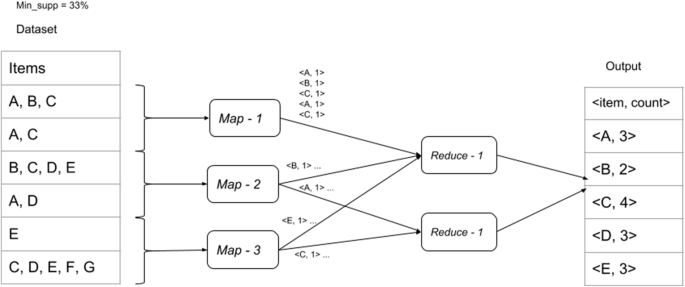Fig. 3

#### The second MapReduce job

In the second MapReduce job, we generate the frequent 2-itemsets. The input for the second MapReduce job is the frequent 1-itemset from the first MapReduce job as well as the transactions database. The frequent 1-itemset is read in from the distributed cache. Paralleling the first MapReduce job, the 2-item (key, value) pairs are generated in the map phase. Then the 2-item (key, value) pairs are passed to the reduce phase of the second MapReduce job. Again, paralleling the first MapReduce job, the reducer then takes these pairs and sums up the values of the respective keys. Reducers will output (item, total_count). Total_count is then compared with min_supp and those equal to or above the min_supp threshold will be kept as the frequent 2-itemset. The frequent 2-itemset and their respective support values are then written to distributed cache. Figure 4 presents the second MapReduce job diagramatically.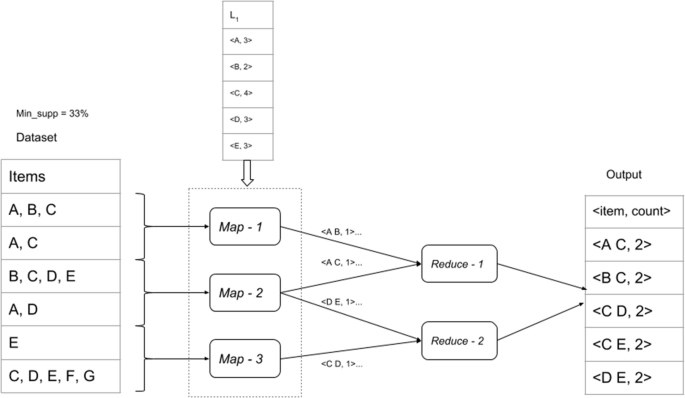Fig. 4

There would be as many similar MapReduce jobs as the number of itemsets required.

#### The third MapReduce job

The third MapReduce job is a map only operation. The output from MapReduce job 2 (frequent 2-itemsets) is read into MapReduce job 3 from the distributed cache. In MapReduce job 3, the confidence and lift are calculated for the frequent 2-itemsets to determine the positive as well as negative association rules. Figure 5 presents the third MapReduce job diagramatically.Fig. 5

#### Determining the positive and negative association rules

If the confidence of (A  B) is greater than the minimum confidence threshold and the lift of (A  B) is greater than 1, than this is a positive association rule. If the confidence of either (A and not B) or (not A and B) or (not A and not B) is greater than the minimum confidence threshold and the lift of this same rule is also greater than 1, then this is a negative association rule. This is presented in Algorithm 1, taken from .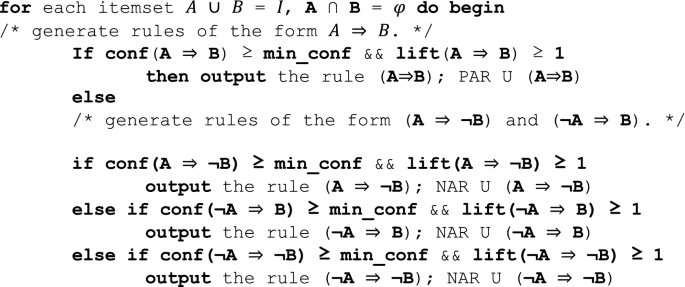Algorithm 1

## Dataset and system parameters used

This section presents the dataset and system parameters used for this study.

### Description of dataset

The WebDocs dataset, publicly available and downloadable from the FIMI website (http://fimi.uantwerpen.be/data/), was used for this study. A description of this dataset can be found in . This 1.5 GB real-life transactional dataset was built from a spidered collection of 1.7 million web html documents mainly written in English, by filtering the documents, removing the html tags and common stop words, and then applying a stemming algorithm. After this process, each document was converted into a distinct transaction containing a set of all distinct terms (items) that appeared in the document. The dataset contains 1,692,082 transactions with 5,267,656 distinct items. The maximal length of a single transaction is 71,472. We replicated and combined the dataset to make bigger datasets of 6 GB, 12 GB and 18 GB for use in our experiments.

### System parameters used

For our experiments, Amazon AWS EMR was used. The EC2 instance type used was c4.xlarge. The Type C instance was chosen because this is a compute optimized type instance in AWS. It has 4 vCPUs and 7.5 GB of RAM.

## Results and discussion

We ran the algorithm at different support and confidence levels, mainly to determine the number of rules generated and to gauge what reasonable support and confidence values would be for this algorithm in the context of big data. Then, to optimize job configuration parameters on Hadoop, we looked at the effect of the following on run time: (i) varing the number of DataNodes; (ii) running the apriori algorithm with and without a combiner; and, (iii) changing the block size.

### Varying minimum support

To estimate the best support and confidence values to be used for the experiments, the experiments were run at different support values, keeping the confidence constant at 95%. For this set of experiments, the original dataset of 1.5 GB, 1 master node and 5 slave nodes, and the default block size of 64 MBs was used. Our algorithm was run for minimum support levels of 15%, 20%, 30% and 40%. Figure 6 presents the number of rules (positive as well as negative) at different minimum support levels. The results show that there are more rules at lower support values.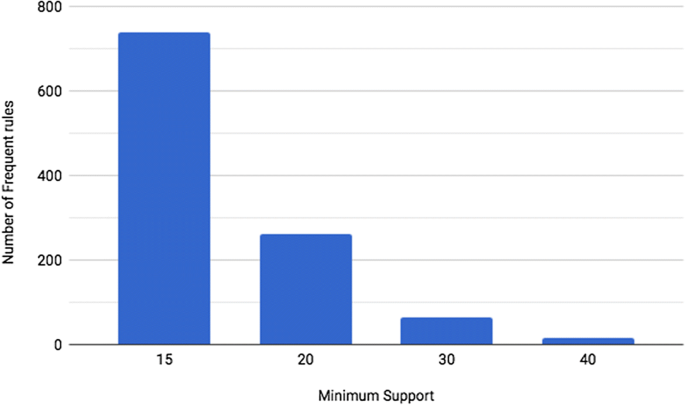Fig. 6

### Varying minimum support and confidence

Next we ran the algorithm for different support levels (15%, 20%, 30% and 40%) at different confidence levels (75%, 85%, 95% and 99%). The original dataset of 1.5 GBs, 1 master node and 5 slave nodes, and the default block size of 64 MBs was used. Figure 7a presents the positive association rules and Fig. 7b presents the negative association rules at the various support and confidence levels.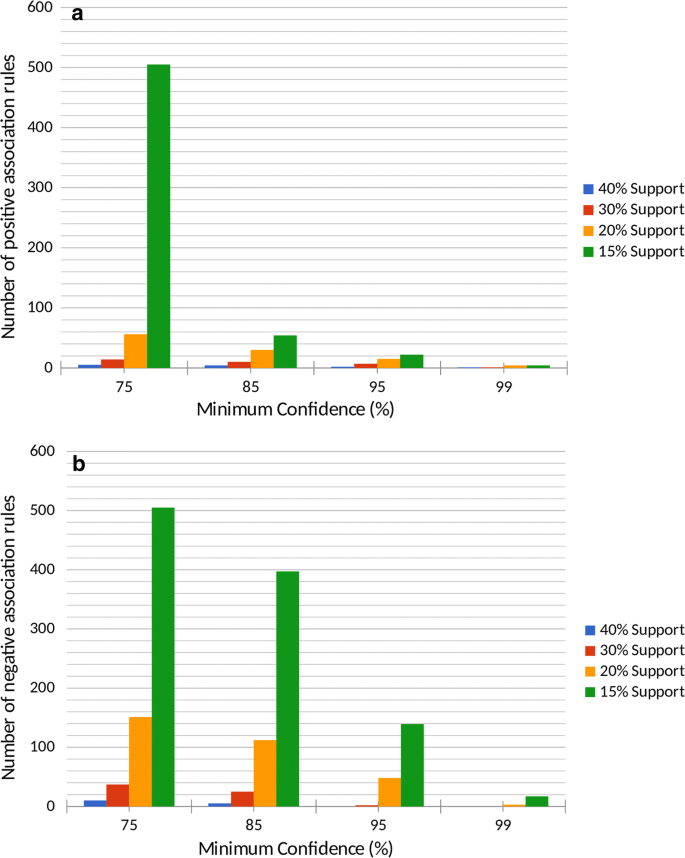Fig. 7

There are more rules for the lower support and lower confidence levels. The results from Figs. 6, 7a, b show that, in the context of big data, there are very few rules at confidence levels greater than 95% and support lower than 40%. And from Fig. 7a, b we can see there were actually more negative rules generated than positive rules (except for minimum confidence of 75%). This means that if we mine just positive association rules, we could be losing a lot of information.

### Varying the number of nodes

In Hadoop, a slave node is where data is stored and where processing takes place. Keeping the master node constant at 1, the number of slave nodes were varied from 5 to 25 at increments of 5. The effects on run-time were recorded. The block size was left at the default of 64MBs.

Figure 8 presents the time required for different numbers of nodes for different data sizes (1.5 GB, 6 GB, 12 GB, and 18 GB) at a minimum support of 40% and minimum confidence of 85%. From Fig. 8 it appears that 25 slave nodes had the optimum performance for all the data sizes. We did not run it for more than 25 slave nodes since we felt that the performance had started leveling off at 25 slave nodes.Fig. 8

### Varying the block size

Block size is a unit of work in Hadoop’s file system. Every read and write operation in Hadoop’s file system is done in multiples of the block size, and the default block size is 64 MB. Blocks allow very large files to be split across and distributed over many machines at run time, allowing for more efficient distributed and parallel processing. A smaller block size will allow for more parallel processing, but there will be the overhead of managing the larger number of blocks, but at the same time, using a larger block size will reduce the amount of parallel processing. So finding the ideal block size is a very important parameter that can be tuned to improve performance.

In the next set of experiments, we varied the block sizes from 32 to 256 MB and recorded the time it took to run the algorithm for the various block sizes for the different data sizes. Figure 9 displays the time required for the different block sizes at minimum support of 30% and minimum confidence of 95%. The number of slave nodes was kept constant at 25. From Fig. 9 it appears that the block size of 256 MB was the most efficient for all data sizes except for the smallest data size of 1.5 GB. The block size of 32 MB had the highest run time for all the data sizes, hence was the least efficient, mainly due to the overhead of managing the larger number of blocks.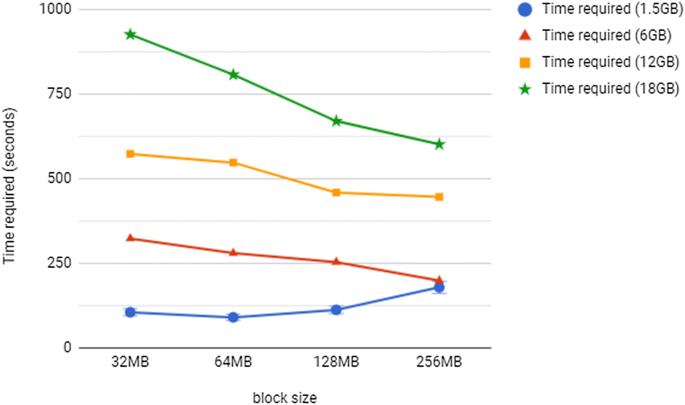Fig. 9

The system used a different number of mappers for the different block sizes for the various data sizes. The number of mappers used for each block size for each data size is presented in Table 1. It can be noted that the number of mappers steadily decreased as the block sizes were increased. The most efficient block size of 256 MB used the lowest number of mappers for all data sizes. The block size of 32MBs would have too many mappers to keep track of.

### With and without a combiner

In MapReduce, the combiner is an optimization function that reduces the amount of data shuffled between the mappers and reducers. Hadoop allows users to specify a combiner function to be run on the map output. The combiner function’s output becomes the input of the reducer function. Combiners are usually more helpful if the MapReduce jobs have limited bandwidth available on the cluster.

So, to experiment with the combiner, the key value pairs from the map phase of the MapReduce jobs 1 and 2 respectively were entered into the combiner. The combiner will pair like keys and calculate the local sum of the values for each key. The combiner output will be in the form (item, list(count, count,…, count)), where items will be from the 1-itemset, 2-itemset, 3-itemset and so on.

The following runs were performed by keeping the minimum support constant at 30% and the minimum confidence at 95%. We recorded the time required to run the full algorithm with and without a combiner for various number of nodes, at the default block size of 64 MB. From Fig. 10a–d, we can see that all the runs with the combiner took less time.Fig. 10

## Conclusion

In this paper, we presented a Hadoop implementation of the Apriori algorithm to mine positive as well as negative association rules. We performed experiments that showed that, for this dataset, there were more negative association rules than positive association rules, so if we mine just for positive association rules, we could be losing some information. Also, for this dataset, 25 slave nodes with a block size of 256 MB gave the best runtime performance. So, for large datasets, a larger amount of parallelization, that is, more number of slave nodes with a higher block size, is more efficient.

## Availability of data and materials

The data was taken from: http://fimi.uantwerpen.be/data/.

## Abbreviations

HDFS:

Hadoop’s Distributed File System

## References

1. 1.

Aggarwal CC, Yu PS. Mining associations with the collective strength approach. IEEE Trans Knowl Data Eng. 2001;13(6):863–73.

2. 2.

Aggarwal CC, Yu PS. A new framework for item-set generation. In: Proceedings of the seventeenth ACM SIGACT- SIGMOD-SIGART symposium on principles of database systems, PODS’98. 1998. p. 18–24.

3. 3.

Agrawal R, Imielinski T, Swami A. Mining association rules between sets of items in large databases. In: ACM SIGMOD conference. New York City: ACM Press; 1993. p. 207–16.

4. 4.

Agrawal R, Srikant R. Fast algorithms for mining association rules. In: VLDB 1994 proceedings of the 20th international conference on very large data bases. 1994. p. 487–99.

5. 5.

Antonie M-L, Zaïane OR. Mining positive and negative association rules: an approach for confined rules. In: Proc. of PAKDD. 2004. p. 27–38.

6. 6.

Antonie L, Li J, Zaiane OR. Negative association rules. In: Frequent pattern mining. Berlin: Springer; 2014. p. 135–45.

7. 7.

Bagui S, Just J, Bagui S. Deriving strong association mining rules using a dependency criterion, the lift measure. Int J Data Anal Tech Strat. 2009;1(3):297–313.

8. 8.

Bala PK. A technique for mining negative association rules. In: Computer2009, Bangalore, India, 2009. p. 1–4.

9. 9.

Brin S, Motwani R, Silverstein C. Beyond market basket: generalizing association rules to correlations. In: Proc. SIGMOD. 1997. p. 265–76.

10. 10.

Cornelis C, Yan P, Zhang X, Chen G. Mining positive and negative association rules from large databases. In: Proc. of CIS. 2006. p. 1–6.

11. 11.

Dean J, Ghemawat S. MapReduce: simplified data processing on large clusters. ACM Commun. 2008;51:107–13.

12. 12.

Gates A, Natkovich O, Chopra S, Kamath P, Narayanam S, Olston C, Reed B, Srinivasan S, Srivastava U. Building a high-level dataflow system on top of MapReduce: the Pig experience. Proc Very Large Data Bases. 2009;2(2):1414–25.

13. 13.

Ghemawat S, Gobioff H, Leung S. The Google file system. In: Proceedings of ACM symposium on operating systems principles, Lake George, NY. 2003. p. 29–43.

14. 14.

Han J, Kamber M. Data mining: concepts and techniques. Burlington: Morgan Kaufmann Publishers; 2012.

15. 15.

Jiang H, Luan X, Dong X. Mining weighted negative association rules from infrequent itemsets based on multiple support. In: 2012 international conference on industrial control and electronics engineering. 2012. p. 89–92.

16. 16.

Kishor P, Porika S. An efficient approach for mining positive and negative association rules from large transactional databases. In: 2016 international conference on inventive computation technologies (ICICT). 2016.

17. 17.

Lucchese C, Orlando S, Perego R, Silvestri F. WebDocs: a real-life huge transactional dataset. In: FIMI ‘04, Proceedings of the IEEE ICDM workshop on frequent itemset mining implementations, Brighton, UK. 2004.

18. 18.

Li N, Zeng L, He Q. Parallel implementation of Apriori algorithm based on MapReduce. In: 2012 13th ACIS international conference on software engineering, artificial intelligence, networking and parallel/distributed computing. 2012.

19. 19.

Lin M-Y, Lee P-Y, Hsueh S-C. Apriori-based frequent itemset mining algorithms on MapReduce. In: ICUIMC ‘12 Proceedings of the 6th international conference on ubiquitous information management and communication. 2012.

20. 20.

Lin X. MR-Apriori: association rules algorithm based on MapReduce, In: 2014 IEEE 5th international conference on software engineering and service science. 2014.

21. 21.

Mahmood S, Shahbaz M, Guergachi A. Negative and positive association rules mining from text using frequent and infrequent itemsets. Sci World J. 2014;2014:1–11.

22. 22.

Oweis NE, Fouad MM, Oweis SR, Owais SS, Snasel V. A novel Mapreduce lift association rule mining algorithm (MRLAR) for big data. Int J Adv Comput Sci Appl. 2016;7(3):151–7.

23. 23.

Ramasubbareddy B, Govardhan A, Ramamohanreddy A. Mining positive and negative association rules. In: 5th international conference on computer science and education, Hefei, China, 2018. p. 1403–6.

24. 24.

Riley E. Indirect association rule mining for crime data analysis. EWU Master’s Thesis. 2015.

25. 25.

Sahu AK, Kumar R, Rahim N. Mining negative association rules in distributed environment. In: 2015 International conference on computational intelligence and communication networks. 2015. p. 934–7.

26. 26.

Savasere A, Omiecinski E, Navathe S. Mining for strong negative associations in a large database of customer transactions. In: Proc. of ICDE. 1998. p. 494–502.

27. 27.

Singh S, Garg R, Mishra PK. Review of Apriori based algorithms on MapReduce framework. In: International conference on communication and computing, ICC 2014, Bangalore, India. 2014.

28. 28.

Swesi IMAO, Bakar AA, Kadir ASA. Mining positive and negative association rules from interesting frequent and infrequent itemsets. In: 2012 9th international conference on fuzzy systems and knowledge discovery. 2012. p. 650–5.

29. 29.

Teng W-G, Hsieh M-J, Chen M-S. On the mining of substitution rules for statistically dependent items. In: Proc. of ICDM. 2002. p. 442–9.

30. 30.

Teng W-G, Hsieh M-J, Chen M-S. A statistical framework for mining substitution rules. Knowl Inf Syst. 2005;7(2):158–78.

31. 31.

Thiruvady DR, Webb GI. Mining negative rules using GRD. In: Proc. of PAKDD. 2004. p. 161–5.

32. 32.

White T. Hadoop: a definitive guide. Sebastopol: O’Reilly Publishers; 2012.

33. 33.

Wu X, Zhang C, Zhang S. Efficient mining of both positive and negative association rules. ACM Trans Inf Syst. 2004;22(3):381–405.

34. 34.

Yuan X, Buckles BP, Yuan Z, Zhang J. Mining negative association rules. In: Proc. of ISCC. 2002. p. 623–8.

Download references

## Acknowledgements

This work has been partially supported by the Askew Institute of the University of West Florida.

## Funding

This work was not funded.

## Author information

Authors

### Contributions

This work was mainly conceptualized by SB and PCD. The programming was mainly done by PCD. Both authors participated in the write-up of the paper. Both authors read and approved the final manuscript.

### Authors’ information

Dr. Sikha Bagui is Professor and Askew Fellow in the Department of Computer Science, at The University West Florida, Pensacola, Florida. Dr. Bagui is active in publishing peer reviewed journal articles in the areas of database design, data mining, BigData, pattern recognition, and statistical computing. Dr. Bagui has worked on funded as well unfunded research projects and has over 45 peer reviewed publications. She has also co-authored several books on database and SQL. Bagui also serves as Associate Editor and is on the editorial board of several journals.

Mr. Probal Chandra Dhar completed his Master’s in Computer Science at the University of West Florida, Pensacola, USA. Mr. Dhar’s research interest includes data mining, Big data and database. He has worked with large-scale distributed systems and worked with big data technologies such as Hadoop and Apache Spark. Currently, he is working at Apple Inc. as a Software Engineer where he is working with a product which is impacting millions of users over the world.

### Corresponding author

Correspondence to Sikha Bagui.

## Ethics declarations

### Competing interests

The authors declare that they have no competing interests.

## Additional information

### Publisher's Note

Springer Nature remains neutral with regard to jurisdictional claims in published maps and institutional affiliations.

## Rights and permissions

Open Access This article is distributed under the terms of the Creative Commons Attribution 4.0 International License (http://creativecommons.org/licenses/by/4.0/), which permits unrestricted use, distribution, and reproduction in any medium, provided you give appropriate credit to the original author(s) and the source, provide a link to the Creative Commons license, and indicate if changes were made.

Reprints and Permissions